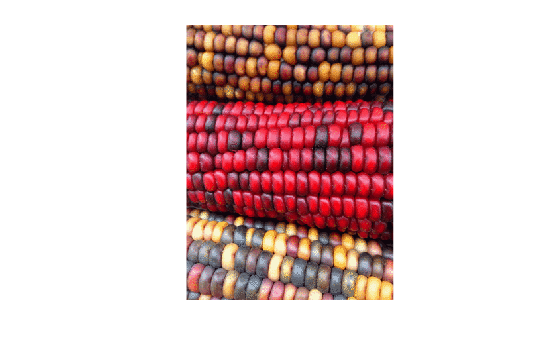Main Content

# ind2rgb

Convert indexed image to RGB image

## Syntax

``RGB = ind2rgb(X,map)``

## Description

example

````RGB = ind2rgb(X,map)` converts the indexed image `X` and corresponding colormap `map` to RGB (truecolor) format.```

## Examples

collapse all

Read the first image in the sample indexed image file, `corn.tif`.

`[X,cmap] = imread('corn.tif');`

The indexed image `X` is a 415-by-312 array of type `uint8`. The colormap `cmap` is a 256-by-3 matrix of type `double`, therefore there are 256 colors in the indexed image. Display the image.

`imshow(X,cmap)`Convert the indexed image to an RGB image. The result is a 415-by-312-by-3 array of type `double`.

`RGB = ind2rgb(X,cmap);`

Check that values of the RGB image are in the range [0, 1].

`disp(['Range of RGB image is [',num2str(min(RGB(:))),', ',num2str(max(RGB(:))),'].'])`
```Range of RGB image is [0.0078431, 0.97647]. ```

## Input Arguments

collapse all

Indexed image, specified as an m-by-n matrix of integers.

• If you specify `X` as an array of integer data type, then the value 0 corresponds to the first color in the colormap `map`. For a colormap containing c colors, values of image `X` are clipped to the range [0, c-1].

• If you specify `X` as an array of data type `single` or `double`, then the value 1 corresponds to the first color in the colormap. For a colormap containing c colors, values of image `X` are clipped to the range [1, c].

Data Types: `single` | `double` | `uint8` | `uint16`

Colormap associated with indexed image `X`, specified as a c-by-3 matrix with values in the range [0, 1]. Each row of `map` is a three-element RGB triplet that specifies the red, green, and blue components of a single color of the colormap.

Data Types: `double`

## Output Arguments

collapse all

RGB image, returned as an m-by-n-by-3 numeric array with values in the range [0, 1].

Data Types: `double`

## See Also

### Topics

Introduced before R2006a

Download ebook